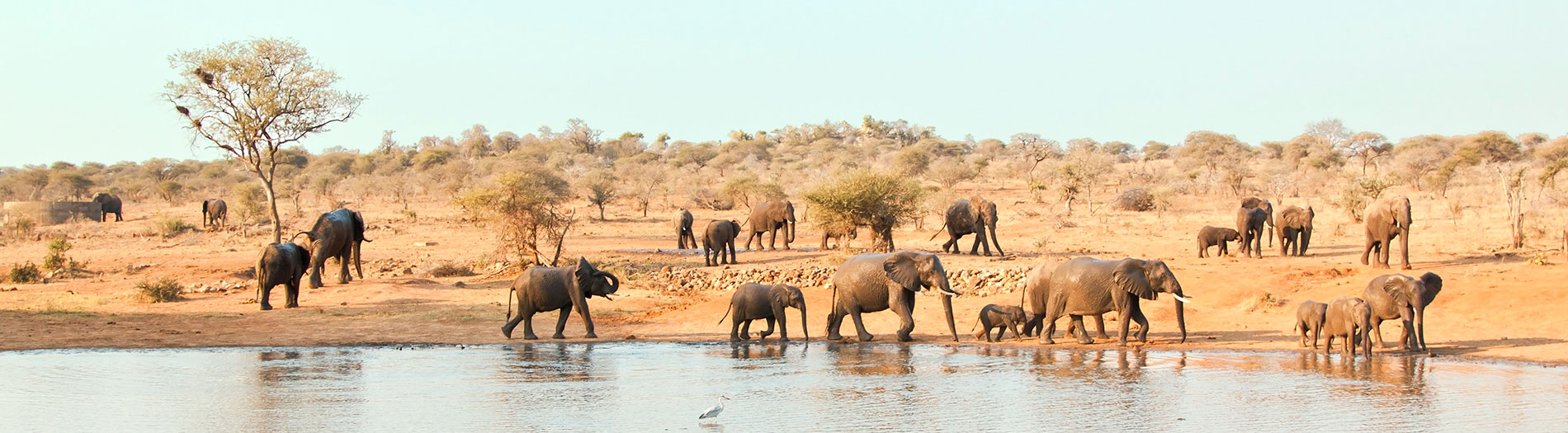### Population Dynamics

These pages present a number of simple population models for exploring basics of population dynamics. You can change initial parameters such as starting population sizes, growth rates and similar then see how they affect population sizes over time. More detail about each model is given on the relevant page. These are great for exploring "what if" scenarios, e.g. what if the population's birth rate went down, or what would happen if predators became more efficient at capturing their prey? I strongly encourage you to experiment freely to see what happens. The results aren't always what you might expect.

Here is one set of guided activities that I have used with these models. You may freely use or adapt these as you see fit for non-commercial purposes. The activties are in Microsoft Word format.

It is not necessary to understand the mathematics behind these models to be able to work with them. Nor is it my intention to go into depth about the mathematics here. The following is a very brief explanation of the basis of these models. Interested readers can readily find more depth with a simple web search.

The exponential growth model is a simple model which calculates the new population in each time interval by multiplying the previous population by the growth rate:

pn = p * r

...where pn is the new population, p is the population size in the previous time period, and r is the growth rate. In this model, a growth rate of 1 means the population is not changing. A population that doubles every time period will have a growth rate of 2. A population that increases by 10% each time period will have a growth rate of 1.1, and so on. Note that the value of p here is always between 0 and 1. An arbitrary multiplier has been used to convert to more realistic numbers in these pages.

The logistic growth model is similar to the exponential model except that it introduces a term that results in a larger percentage of the population dying (or emigrating) as the population size increases.

pn = p * r * (1 - p)

...where (1 - p) is the percentage of the new population that survives. Notice how, as p gets larger, (1 - p) gets smaller, i.e. a smaller percentage of the population survives. This represents the increasing effects of limiting factors on the population as it nears its carrying capacity.

The various predator-prey models are based on variations of the Lotka-Volterra equations, a system of differential equations that describe the interaction between two species, one of which preys on the other. Wikipedia has a description of these equations here.

All images are licensed stock images.